# HSC Maths Ext2 - Complex Numbers - Solving Quadratic Equations with Complex Coefficients

##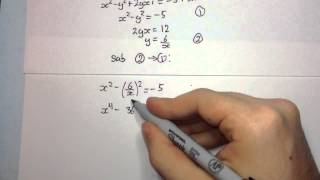By Mathema Tutoring

Welcome to my HSC 4 Unit maths: Complex Numbers series. In this video we learn how to solve quadratic equations with complex coefficients. Be sure to SUBSCRIBE for more videos in the future.# Solving matrix equations

##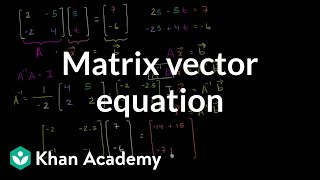By Khan Academy

After he represented a system of equations with a single matrix equation, Sal solves that matrix equation using the inverse of the coefficient matrix.# Parabola intuition 1

##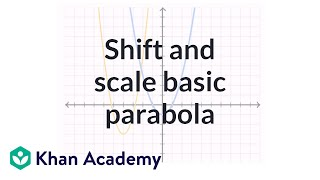By Khan Academy

Sal solves an example task where he adjusting the coefficients of a quadratic equation in a way that shifts and stretches the parabola corresponding to that equation.# Different ways to solve equations

##By MathPlanetVideos

Solve the following expression 4x−7=x/3+4# Solving Quadratic Equations with Complex Solutions (N-CN.8)

##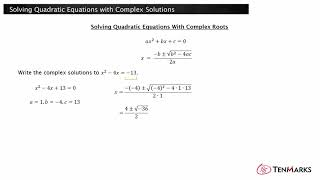By TenmarksAmazon

This lesson explains how to solve quadratic equations with real coefficients that have complex solutions.# Inverse Laplace Examples

##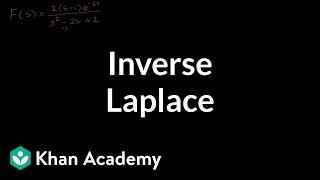By Khan Academy

This video lecture series on Differential Equations includes Introduction to Differential Equations Separable Differential Equations Exact Equations Integrating Factors First Order Homogenous Equations Second Order Linear Homogenous Differential Equations Complex Roots of the Characteristic equations Undetermined Coefficients Laplace Transform.....# 2nd Order Linear Homogeneous Differential Equations 4 | Khan Academy

##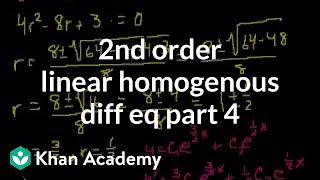By Khan Academy

This video lecture series on Differential Equations includes Introduction to Differential Equations, Separable Differential Equations, Exact Equations, Integrating Factors, First Order Homogenous Equations, Second Order Linear Homogenous Differential Equations, Complex Roots of the Characteristic equations, Undetermined Coefficients, Laplace Transform.....# Solving matrix equations

##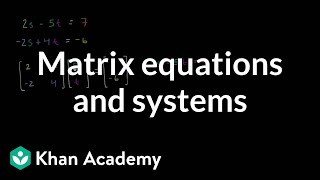By Khan Academy

Sal shows how a system of two linear equations can be represented with the equation A*x=b where A is the coefficient matrix, x is the variable vector, and b is the constant vector.# Writing systems of equations as matrix equations

##By Khan Academy

Sal shows how a system of two linear equations can be represented with the equation A*x=b where A is the coefficient matrix, x is the variable vector, and b is the constant vector.# Solve equations and simplify expressions

##By MathPlanetVideos

Solve the given equation 12(3b−b4a)=36# Solve equations with two rational expressions

##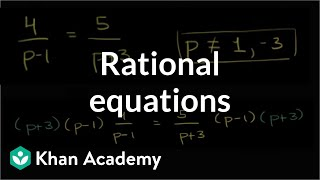By Khan Academy

Sal solves the equation 4/(p-1)=5/(p+3).# Solve equations with one rational expression (advanced)

##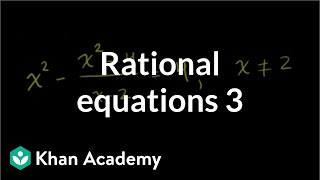By Khan Academy

Sal solves the following equation by first simplifying the rational expression: x^2-(x^2-4)/(x-2)=4.# Solve equations with one rational expression (advanced)

##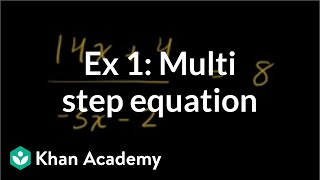By Khan Academy

Sal solves the equation (14x+4)/(-3x-2)=8.# Solve equations with two rational expressions

##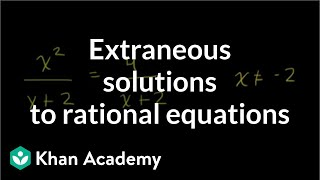By Khan Academy

Sal solves the following equation and eliminates the resulting extraneous solution: (x^2)/(x+2)=4/(x+2).# Solve equations with two rational expressions

##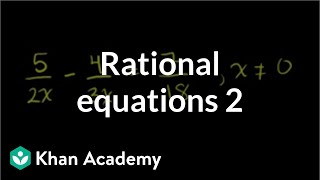By Khan Academy

Sal solves the equation 5/(2x)-4/(3x)=7/18 by first finding the LCM (least common multiple) of 2x and 3x.# AP Stats 7.2 Correlation and r value... what is it? finding on TI intro

##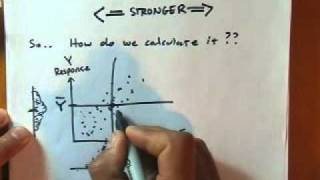By MrNystrom

S-ID.8: what is the r value the correlation coefficient Here is a quick explanation of the r value and what it means.. How to find r value and equation on TI 84 83 89 .. r value basics# Solving Quadratic Equations by Factoring - MathHelp.com

##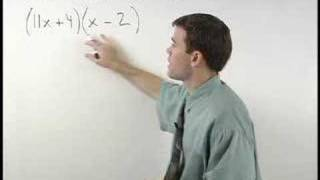By yourteachermathhelp

This video is a clip of a smaller segment. Students learn to solve quadratic equations by the method of their choice, using the following rules. If possible, use the factoring method. If there is no coefficient on the squared term, and the middle term of the trinomial is even, use completing the square. If there is a coefficient on the squared term, and/or if the middle term is odd, use the quadratic formula. If the variable only appears in the squared term, get the variable by itself on one side of the equation and square root both sides.# Solve equations with one rational expression (basic)

##By Khan Academy

Sal solves the equation (14x+4)/(-3x-2)=8.# Standard Form of a Line | MathHelp.com

##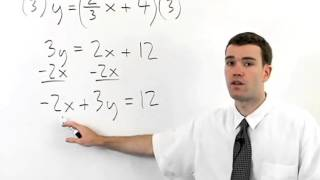By MathHelp.com# Simultaneous Equations - Example to solve 1

##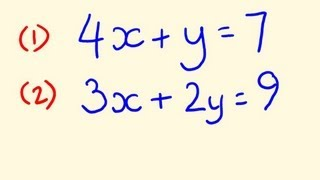By tecmath